# Unit vector facts for kids

Kids Encyclopedia Facts

A unit vector is any vector that is one unit in length.

Unit vectors are often notated the same way as normal vectors, but with a mark over the letter (e.g.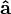$a-hat$ is the unit vector of a.)

To make a vector into a unit vector, divide it by its length: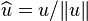$\widehat{u} = u / \lVert u \rVert$

## In component form

Three common unit vectors used in component form are$i-hat$,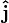$j-hat$ and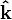$k-hat$, referring to the unit vectors for the x-, y- and z-axes respectively. They are commonly just notated as i, j and k.

They can be written as the following: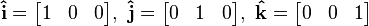$Components i,j and k$

## Images for kidsUnit vector Facts for Kids. Kiddle Encyclopedia.